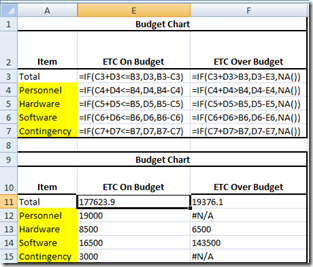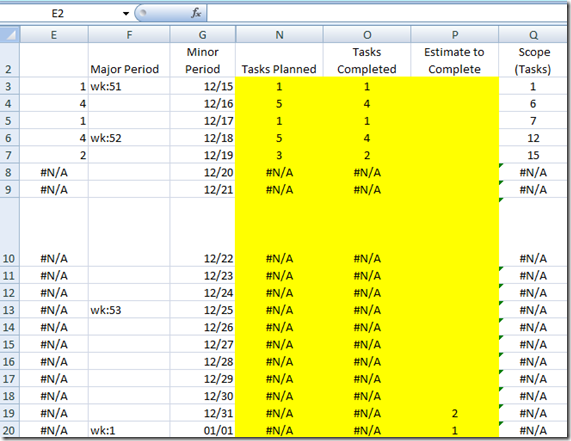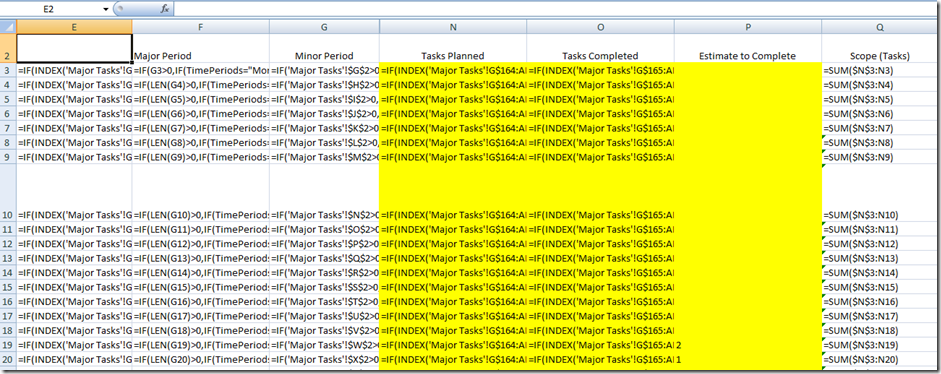# How-to Show All Formulas in Excel SpreadsheetHave you ever wondered which cells have actual values entered and which ones are calculated formulas in your Excel spreadsheet?  Perhaps you are auditing a spreadsheet or checking your formulas and you need to see if any of the formulas are different than others.How do I show my formulas in a spreadsheet?

You can show your formulas in a worksheet by pressing the following key strokes:

CTRL + ~

So if your worksheet looks like this:Hold the Control Key and then press the ~ key (CTRL + ~).

The ~ is typically left of the 1 on most keyboards above the ‘ (single left quote key)

Now your Excel worksheet will show all formulas like this:To toggle back to see values instead of formulas, simply press CTRL + ~ again.